SYLLABUS  Previous: 3.3.1 Wiener process and  Up: 3.3 Improved model using  Next: 3.3.3 Evaluate an expectancy

3.3.2 Itô lemma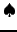[ SLIDE Rules - Ito's lemma || VIDEO modem - LAN - DSL]

Despite the short-hand differential notation that has been used so far, the stochastic differential equation (3.3.1#eq.1) is formally defined only in its integral form: in other words, a probability weighted average has to be carried out before the random sampling from the Wiener process acquires any significance. The stochastic or Itô calculus dealing properly with the extensions of the usual Riemann integrals to non-smooth differentials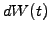leads to the Itô lemma and draws on mathematics that goes beyond the scope of this course. The same result can however be derived from a Taylor expansion in multiple dimensions, keeping terms up to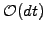and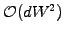and applying the special rules for stochastic calculus: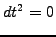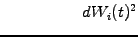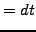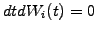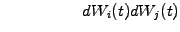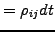(3.3.2#eq.1)

After substitution of the value of the stochastic differential (3.3.1#eq.1), this leads directly to Itô's lemma, here given for the function of only one stochastic variable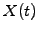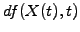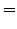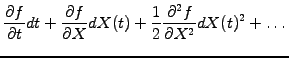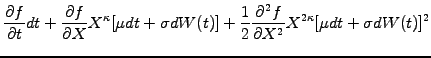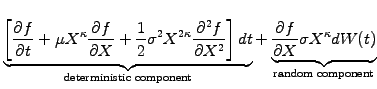(3.3.2#eq.2)

In words, the Itô lemma states that the differential of a stochastic function is the superposition of a deterministic component proportional to the time step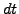, and a random component proportional to the Wiener increment. Remember that the factor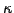= 0 or 1 here chooses between a normal or log-normal distribution of the price increments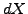and can also take other values if this is found to be appropriate.

SYLLABUS  Previous: 3.3.1 Wiener process and  Up: 3.3 Improved model using  Next: 3.3.3 Evaluate an expectancy